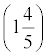##### 2022 / 2023 ASVAB For DummiesYou will need to know some forms of measurement and how to perform conversions for the ASVAB. Because science is based on developing objective facts — evidence and results that are measurable and experiments that can be reproduced — measurements are an important part of science. And because this subtest is all about science, you can expect to run into a few questions about measuring scientifically on the ASVAB.

## Doing the metric thing

The metric system (or SI, the International System of Units) is based on a decimal system of multiples (and fractions) of ten. Scientists almost always use the metric system for precise measurement. No, they don’t use it just to make the ASVAB harder for you; they use this system so a standard exists among scientists around the world. In fact, the majority of countries around the globe use the metric system — the United States is in its own world when it comes to the Imperial (non-metric) system.

Here are some units of measurement you need to know for the General Science subtest of the ASVAB:

• The meter (m) is the unit of length.
• The liter (L) is the unit of volume.
• The gram (g) is the unit of mass (similar to weight).
You can attach prefixes to these base units to indicate units that are larger or smaller. Check out these metric prefixes.
 Prefix Symbol What It Means milli- m One-thousandth (0.001) centi- c One-hundredth (0.01) deci- d One-tenth (0.1) deca- da 10 hecto- h 100 kilo- k 1,000 mega- M 1,000,000
Here are some abbreviations of common metric measurements.
 Length Liquid Volume Mass millimeter (mm) milliliter (mL) milligram (mg) centimeter (cm) centiliter (cL) centigram (cg) meter (m) liter (L) gram (g) kilometer (km) kiloliter (kL) kilogram (kg)

## Figuring temperature conversions

When you think of temperature, you may think of the Fahrenheit and Celsius scales, which measure temperatures in degrees. Scientists actually use three different scales to report temperature:
• Fahrenheit (ͦ F): This scale is more common in the United States. On the Fahrenheit scale, water freezes at 32 ͦ F and boils at 212 ͦ F.
• Celsius or Centigrade (ͦ C): This scale is the metric standard worldwide. On the Celsius scale, the freezing point for water is 0 ͦ C, and the boiling point for water is 100 ͦ C).
Kelvin (K): Scientists have theorized that the coldest anything can get is –273.15 ͦ C. They believe that at this temperature, molecular motion would stop. That’s pretty darn cold! This temperature, often called absolute zero, is assigned to be 0 on the Kelvin scale (with the units the same size as degrees on the Celsius scale). On this scale, the freezing point of water is 273.15 K, and the boiling point is 373.15 K.

The word degrees isn’t used when stating temperature in kelvins. Scientists who work with thermodynamics, such as physicists and astronomers, measure temperature using kelvins. For instance, the surface temperature of planets is always stated in kelvins.

An ASVAB question may ask you to convert temperatures from one scale to another, so here are some formulas to commit to memory (C stands for the temperature in degrees Celsius, and F is the temperature in degrees Fahrenheit):

To convert from Celsius to Fahrenheit, use this formula:

To convert from Fahrenheit to Celsius, use the following formula:

To get temperatures in the Kelvin scale, add 273.15 degrees to the Celsius temperature:

To go from kelvins to degrees Celsius, do the opposite: Subtract 273.15 from the kelvin temperature. Then you can convert the Celsius temperature to Fahrenheit if you like.

Here’s a quick temperature conversion system that may be easier to remember. (Note: This process only works with Celsius and Fahrenheit.)

Add 40 to the temperature you want to convert.

Multiply this sum by 5/9 if converting from Fahrenheit to Celsius or 9/5 if converting from Celsius to Fahrenheit.

Subtract the 40 you added at the beginning to yield the result.

An easy way to remember whether to use 9/5 or 5/9 in the conversion is to associate the f in Fahrenheit with Fraction (5/9 is a proper fraction); similarly, 9/5 can be converted to a mixed number:C is for Convert and Celsius.